﻿ Design of a Picture-seeing and Talking System Based on Attention MechanismPublications are Open
Access in this journal
Article Versions
Export Article
• Normal Style
• MLA Style
• APA Style
• Chicago Style
Research Article
Open Access Peer-reviewed

### Design of a Picture-seeing and Talking System Based on Attention Mechanism

Kexin Ye , Lei Zhang, Tianran Li
American Journal of Electrical and Electronic Engineering. 2022, 10(1), 6-23. DOI: 10.12691/ajeee-10-1-2
Received June 12, 2022; Revised July 17, 2022; Accepted July 27, 2022

### Abstract

In order to solve this problem, this paper proposes an image title generation model based on deep loop architecture. This model combines some new achievements in computer vision and machine translation, and can generate natural sentences that accurately describe the image for an uncomplicated physical image. The model is trained to maximize the accuracy of the target description sentence in a given training image. The training data set was mainly completed by the MSCOCO data set, and in the later adjustment stage, some feature pictures I specifically looked for on the Internet were included for improvement. Test experiments on several data sets verify that the model has the ability of basic accurate image description. This model is usually more accurate in the case of uncomplicated physical pictures, which I have verified both qualitatively and quantitatively. The final result can input a qualified image and output a natural language sentence to describe the main content of the image.

### 1. Introduction

1.1. Research Background

"Image captioning" (image captioning), as the name suggests, is to output a sentence or a set of keywords describing the image by inputting an image, requiring the sentence to be fluent and the tense accurate. For the human beings in this article, looking at pictures and talking is the simplest thing, and children from kindergarten began to practice reading and talking 1. But for computers, this task is very difficult and challenging. Because we want the computer to realize the function of looking at pictures and talking, on the one hand, it requires the machine to understand the image - to be able to recognize the scenes and things in the image, and on the other hand, it also requires the machine to realize the language organization ability similar to the human - with a reasonable language to express the content of the image it understands. The image below is an example of three images and their corresponding descriptions. In this paper, we can regard reading and speaking as a translation task, but it is not the language-to-language translation that we are familiar with, but the translation from image to language. Speaking of translation, we have to mention the application of machine translation (Machine Translation) - output a literal sentence for the input sentence you give. The latter can be the expression of the former in other languages, that is, a translation in the true sense, or it can be a combination of keywords of the former, that is, an abstract. As the so-called "collision produces sparks", image-to-language translation can also learn from language-to-language translation in machine translation, so many related researches on image caption generation can be regarded as machine translation technology in the field of NLP. Expansion and innovation in the field of CV. And gradually formed the research direction of looking at pictures and speaking based on the attention model. Before deep learning was discovered and used, almost all machine translation algorithms were implemented using mathematical and statistical methods. With the development of deep learning, various models based on deep learning technology have gradually begun to be widely used in the field of NLP, including the field of natural machine translation 1. Since 2014, the "Sequence to Sequence" (Seq2Seq) model based on deep learning networks has gradually become the mainstream method for machine translation in the field of NLP. In 2015, the attention mechanism appeared and was added to the Seq2Seq model, which overcomes many major problems of the basic Seq2Seq model and greatly improves the quality of machine translation.

1.2. Research Significance

Create an attention-based image caption generation model that enables the program to generate a caption that matches the content of a given image--that is, complete the image description. The essence of the problem of image caption generation is the problem of changing from vision to language, and the explanation is very simple: look at the picture and talk. Just as kindergarten teachers hope that children can complete the task of looking at pictures and talking, this paper also hopes that the algorithm can give a fluent sentence that can describe the content of the image according to the input image.

Image description solves the problem of automatically obtaining the corresponding descriptive text after a given image. It is an intersection of computer vision, natural language processing and machine learning, and it is also a very challenging artificial intelligence research problem. Its application areas are very broad, and can involve various aspects such as recognition, detection, segmentation and pose estimation, semi-supervised, unsupervised, transfer, representation and few-shot learning. It is regarded as a great challenge in the field of artificial intelligence, and the progress of this research has an important guiding and basic role for the development of artificial intelligence in the future.

1.3. Research Status at Home and Abroad

Since the birth of deep learning, many companies and even individuals have been attracted to research in this field. In recent years, works and applications on the direction of deep learning have emerged in an endless stream, and progress has been rapid, and new ideas and works have sprung up like mushrooms after a rain.

Many large companies in the world are also doing research on deep learning. For example, companies such as Apple, Microsoft, Google, Amazon, etc., while conducting research, they also have a lot of works in practical applications, such as Apple's intelligent voice robot "SIRI", and Microsoft's "Xiaoice", and even the well-known first player in Go, “Alpha Dog” 2.

Although the research on deep learning in this country is relatively slow, the current development momentum and speed are catching up with the international trend. Many large companies are gradually getting into this space. For example, domestic companies such as Alibaba, Tencent, Baidu, and Huawei have already joined the research in this field and have achieved considerable results and works. In addition, some small companies are also researching in this area, hoping to expand their technology to use in other industries.

1.4. The Main Research Content of This Topic

The ultimate goal of this topic is to design a complete trained image caption generation system, which can output a corresponding natural language sentence for a qualified input image to describe the main content of the image. This requires the completion of these studies:

Study the contents of deep learning, understand the principles of neural network models and master the application methods.

Learn to master coding in the Python language and build the Pycharm environment.

Use the data set to train a large amount of the designed model, test and analyze the trained model, give a score and analyze the deficiencies, and then continuously improve the image description ability of the model by adjusting the code or special training.

### 2. Research Ideas and Tools

2.1. Research Ideas

In the field of computer vision, the problem of generating natural language descriptions from visual data has always been a hot research topic, but most of them are still mainly for video. This leads to a very complex system, as it is composed of a combination of visual primitive recognizers and structured formal languages. Such as And-Or diagrams or logical systems, which are further transformed into "human languages" through rule-based systems. Most of these systems are artificially designed, the balance ability is relatively fragile, and they have only been used in more limited fields, such as monitoring and sports 3.

The problem of text generation based on natural image captions is also one of the hotspots in recent years. Natural language generation systems are driven by recent advances in recognizing objects, their properties, and locations, but the downside is that their expressiveness is greatly limited and inevitably huge flaws.

Today, most of the work on the problem of ranking descriptions for a given image has been solved. The idea of this approach is to co-record images and text in the same vector space. For the input image, just retrieve the description output similar to the image. More commonly, neural networks co-embed images and sentences to split the image content into many feature values, but do not attempt to generate detailed descriptions 3. All in all, the above methods fail to describe the composition of objects that the system has not "seen", even if the object has been trained on the training data. Furthermore, they lack a process for evaluating the quality of the generated descriptions.

Therefore, in the design of this paper, a deep convolutional network CNN for image classification and a recurrent network RNN for ranking modeling are combined to create a special network for generating image captions. The RNN is trained in this single-input single-output network environment. The difference is that the input provided by this system is an image processed by a convolutional network instead of a sentence. There have been similar models before that use neural networks, with a feed-forward network, to predict the image of the next word and the previous word. A recurrent neural network is then used to accomplish the same prediction task. This is somewhat similar to the idea of this paper, but there are important differences: the paper uses a more comprehensive RNN model and directly provides the RNN's visual input model, which allows it to interpret the objects tracked by the RNN as text. It is because of this difference that our system achieves better results in testing. Finally, a joint multimodal embedding space is constructed using powerful computer vision models and LSTMs 2. As shown in Figure 1 below:

• Figure 1. Schematic diagram of the overall model

This NIC model is based on a neural network consisting of a language of convolutional neural network CNN and recurrent neural network RNN. It can output a complete sentence in natural language that conforms to the meaning of the graph from the input image, as shown in the above example.

2.2. Basic Introduction to Using Model Database
2.2.1. CNN (Convolutional Neural Network)

The origin of Convolutional Neural Networks (CNN) was in the late 1880s and early 1990s. It shines in many fields such as computer vision and natural language processing. It is a feedforward neural network that contains convolutional computation and has deep structure at the same time, and is one of the classic algorithms of deep learning. Convolutional Neural Networks are also known as "Translation Invariant Artificial Neural Networks" 5. In fact, this network has become one of the most influential innovations in computer vision today.

The convolutional neural network is one of many types of neural networks. The difference between it and the traditional neural network is that the matrix multiplication of the latter is replaced by the convolution operation of the former. Through the convolution operation, the convolutional neural network can identify the planar structure that effectively utilizes the input data 4. Therefore, convolutional neural networks have huge advantages over traditional neural networks in the field of image recognition and speech recognition.

Convolutional Neural Networks are now also one of the most studied genres in Deep Learning. The reason for this phenomenon is that it can directly process the original input image data, eliminating the complex preprocessing process. As the name suggests, its core lies in the word "convolution". In the past machine deep learning, features such as BP and HOG can be regarded as a special form of convolution. "Convolution" uses different parameters to The abstract feature described by this method is the most similar to the abstract feature of the original image.

• Figure 2. Working principle diagram of CNN operation

In the above picture, the convolutional neural network uses a sliding window (convolution kernel) to easily "screen" the original picture area, multiply the corresponding pixels one by one, and then accumulate (I*K), the pixel's The convolution results are freshly released. Therefore, people often say that convolution is like a sieve, which will follow certain mathematical operation rules (with the help of convolution kernel "multiply and accumulate") to process the original image twice (actually an integral), so this article uses the following function to represent. This formula: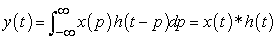(1.1)

Let's take a look at a typical CNN example, for the image processing process with a resolution of 28*28:

• Figure 3. Schematic diagram of image processing by CNN

The Conv in the picture is called the convolution layer (using 5*5 convolution kernel, Step is 1), its main function is to extract the features of the input data; Pool is called the pooling layer, also known as downsampling (Sample), its function is to reduce the complex high-dimensional data; FC is a fully connected layer, and the data in the pooling layer is directly calculated in FC to obtain the result. In this paper, the intermediate layers such as convolutional layers and pooling layers are collectively referred to as hidden layers. Next, take a deep dive into the meaning of each layer to deepen your understanding of CNN.

• Figure 4. Schematic diagram of each layer of CNN

1. Convolutional layer and weight sharing

In biology, neurons process data according to certain rules, and each neuron is closely connected to the previous neuron for feature extraction. As shown in the figure below, we set a total of one million neurons in this paper, so for the complex image processing of 150 pixels, 1012 connections will be established, which creates a huge number of weights, and the calculation process will also becomes very complicated.

• Figure 5. Schematic diagram of the principle of CNN processing the weight problem

How to solve a large number of weight calculation problems? The key is "classification", where each type of neuron corresponds to a set of weights. As shown in the figure above, in this paper, we only use the convolution operation of 10,000 weights to approximately simulate the processing process of neurons. The parameter operation in this process is not complicated for the computer, especially for the efficient convolution. The calculation method is more simple and complete.

2. Activation layer

The activation layer has a masterpiece called a neural network, which is a true reflection of the working mechanism of neurons. The widespread use of the ReLU function in CNN today effectively solves the problem of gradient diffusion, in which case you don't have to care about feature "scatter" and you can even ignore "pre-training". Generally, activation layers are added after convolutional or pooling layers, but there is no clear position definition. If you only need to build a simple neural network, the activation layer is usually not added.

3. Dropout layer

Another big flaw of neural networks - "overfitting", has plagued people for a long time until the Dropout layer was proposed. Corresponding to the under-fitting that caused the "gradient diffusion" problem earlier, the conventional method to solve the "over-fitting" problem is the model averaging method, that is, to avoid it by training multiple networks for weighted combination, but it requires a larger amount of calculation. Problems will follow 5. The Dropout method can solve all problems very well. It was first proposed by Hinton. The principle is that neurons only retain the parameters of the weights and update them in the next training process. And according to a certain random strategy, the neurons in each training are different, so that the neuron nodes can work in turn. This random process is more similar to the human brain.

• Figure 6. Schematic diagram of the working principle of training neurons

Each Dropout process is equivalent to a simplified transformation of the network (as shown in the figure above). The simplified network nodes can participate in the weight update, and Dropout is performed multiple times during the entire training process, so that each node participates in the learning. And training, the process is very simple, but can get excellent results.

Maybe there is not enough rigorous mathematical formula derivation, but no matter from which angle to understand, Dropout is an intuitive and effective method, which originates from the intuitive understanding of biology and has been proved by a large number of experiments.

Why can it converge effectively? What theory is used to avoid getting stuck in local maxima? Maybe the knowledge of these problems is not enough to answer these questions, but sometimes you don't need to struggle, you can solve the problem. As for the detailed and complicated reasons behind it, don't worry too much.

4. Fully connected layer

The fully connected layer can be intuitively understood as a simplified calculation of data. Finally, even omitted.

5. Regression layer

Strictly speaking, the regression layer is an independent part, but it can sometimes be regarded as a piece of the fully connected layer, and its job is just to connect the processes here. Regression is a relatively easy-to-understand concept. The logistic regression and Gaussian regression mentioned above are all types of regression. Simply speaking, it is a process of classifying image features, regardless of the categories. Its essential role is to map a P-dimensional vector to another K-dimensional vector, and its formula is described as follows: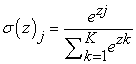(2.2)

Thereby, the probability represented by the corresponding category is obtained, which is the classification result that this article wants.

2.2.2. RNN (Recurrent Neural Network)

Unlike other neural networks, RNN has a special structure. The difference between RNN and DNN and CNN is that its output is not only about the input of the previous moment, but also gives the network a 'memory' function of the previous content. The reason why RNN is called a recurrent neural network is that its output is the result of comprehensive "consideration" of the previous input and all previous outputs 7. The specific working principle is that the network will memorize the previous information and associate it in the calculation of the current output, that is, the nodes between the hidden layers are changed from "isolated" to connected to each other, and the output of the hidden layer not only includes the output of the input layer Also includes the output of the hidden layer at the previous moment 8.

So what is the reason for needing RNN (Recurrent Neural Network)? As mentioned above, ordinary neural networks can generally only process one input individually, and the previous input and output have nothing to do with the next input and output. However, when you are faced with some tasks that need to process sequence information, that is, there is a relationship between the previous input and the subsequent input, you need to use the RNN's ability to process sequences. Let's first look at a simple RNN model as shown in the figure below, which consists of an input layer, a hidden layer and an output layer:

• Figure 7. Schematic diagram of a simple RNN model

It can be analyzed from the figure that x is a vector group, which represents the value of the input layer; s is also a vector group, which represents the value of the hidden layer, U is the weight matrix from the input layer to the hidden layer, and o is also a vector group, which Represents the value of the output layer; the corresponding V is the weight matrix from the hidden layer to the output layer 9.

As can be seen from the figure, the value s of the hidden layer of the recurrent neural network depends not only on the current input x, but also on the value s of the previous hidden layer. The weight matrix W is the last value of the hidden layer as the weight value (proportion value) of this time's input 10. The corresponding specific diagrams are given below:

• Figure 8. Schematic diagram of RNN

As can be seen from the above figure, the hidden layer of the previous time step can affect the hidden layer of the current time step.

Expand the above picture to get the following schematic expansion diagram:

• Figure 9. Schematic diagram of RNN unfolding according to the timeline

This looks very clear. The following formula can be used to specifically express the calculation method of the recurrent neural network: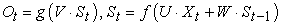(2-3)

Finally, an overview of the RNN is given:

• Figure 10. RNN overview diagram

2.2.3. MSCOCO Dataset

Through comprehensive consideration and multi-party comparison, this paper adopts the MSCOCO data set as the main training and testing data set of the system model in this paper. Therefore, the COCO data set is mainly introduced here, which is a large-scale and rich image captioning. data set. This dataset aims at scene understanding, intercepts images from complex daily scenes, and calibrates the targets in them accurately. The images contain 91 classes of objects, 328,000 images and 2,500,000 labels 11. As of now, COCO is the largest dataset that can be found on the Internet under the condition of semantic segmentation, providing more than 80 categories and more than 330,000 images. 200,000 of them are labeled, and the number of individuals in the entire dataset exceeds 1.5 million. The biggest advantages of this dataset are three: the breadth of detection targets, the discrimination of contextual relationships between targets, and the precise localization of the two-dimensional positions of target features. Its main features are as follows:

1) Target segmentation

2) based on text recognition

3) Multi-target per image

4) More than 300,000 images

5) Over 2 million instances

6) 80 categories

7) Average 5 targets per image

8) There are key points for 100,000 people

An example of MSCOCO data is shown in the figure below.

• Figure 11. Example of MSCOCO data

The COCO dataset has been released twice. The first release was in 2014. It contains 82,783 training samples, 40,504 validation samples, and 40,775 test samples, as well as 270,000 segmented portrait images and 886,000 segmented object images. The second release in 2015 included 165,482 training samples, 81,208 validation samples, and 82,434 test samples. There are several important keys to know about using this dataset:

1. file_name #points to a string, which is the name of the image;

2. height, width #The height and width of the image pointed to;

3. id # points to the label unique to the image. The numbers are not repeated. It can be regarded as the information of the image itself, just like the numbers on the ID card.

4. annotation #Points to a list that contains multiple thesaurus, each title corresponds to an annotated image.

Some examples of annotated images in the coco dataset:

• Figure 12. An example of annotated images in the COCO dataset
2.3. Environment Construction and Libraries
2.3.1. PyCharm

PyCharm is a common modern tool that people often use to improve their productivity when developing in the Python language.In addition, the IDE also integrates some other advanced functions, and the use of Pycharm in professional web development often has unexpected good results.

2.3.2. Anaconda and Libraries

Anaconda is an open source Python distribution that includes Python, conda (a Python package manager), and various packages for scientific computing, which can be used completely independently without additional Python downloads. Using Anaconda has the following benefits:

1) Eliminate differences in system platforms and solve underlying dependencies. After installing a package, all the things that depend on it are dealt with, so you don't have to worry about it.

2) There is a concept of virtual environment. Each environment is isolated, and different Python versions and various packages can be set. It does not conflict with the system, and can be switched at will. If you want to delete it, it is also cleaned up together.

2.3.3. Tensorflow

TensorFlow is a software library that contains various open source code and is often used in high-performance numerical operations. Because of its relatively flexible architectural design, users can use it to deploy computing jobs on various platforms and devices.

TensorFlow uses a data flow graph for computation, so first we need to create a data flow graph in this article, and then place the tensor data in the data flow graph for computation. Nodes represent mathematical operations in the graph, and edges represent multi-dimensional data arrays interconnected between nodes, namely tensors 13. When training a model, tensors flow continuously from one node in the dataflow graph to other nodes. This is where the name TensorFlow comes from. In machine learning, values are usually composed of 4 types:

(1) scalar (scalar): a numerical value, which is the smallest running unit in the calculation process, such as "9" or "567".

(2) Vector: A one-dimensional array composed of some scalars, such as [1, 3.2, 4.6], etc.

(3) Matrix: A two-dimensional array composed of scalars.

(4) Tensor: A data collection composed of multi-dimensional (usually) arrays, which can be understood as a high-dimensional matrix. The data flow diagram of Tensorflow is as follows:

• Figure 13. Tensorflow data flow diagram

The advantages of using TensorFlow are mainly reflected in the following aspects:

(1) The architecture of TensorFlow is very intuitive, it has a "tensor flow". Users can easily and intuitively see all the links of tensor flow.

(2) TensorFlow is suitable for easy deployment on CPU/GPU for distributed computing.

(3) TensorFlow is highly cross-platform and flexible. TensorFlow is not only competent for Linux, Mac and Windows systems, but also performs well on mobile terminals.

TensorFlow also has its shortcomings, mainly because its code is relatively low-level and requires a lot of writing by users. Moreover, for many similar and repeated functions, users have to "rebuild the wheel" 13. However, "the flaws do not hide the flaws", TensorFlow has been sitting on the top of the usage rate of many deep learning frameworks for a long time with its profound technical precipitation and stable performance.

2.3.4. NumPy

NumPy is a module for efficient computation and its analysis.

The main functions of NumPy are:

1. ndarray, a data structure that can save a lot of running memory.

2. Implement fast operation on data without looping.

3. Tools for reading and writing disk data and for manipulating memory-mapped files.

4. Generate new linear functions and pseudo-random numbers, and perform Fourier transform on the target.

5. Tools for integrating C, C++ and other codes.

The dimension of a NumPy array is called the rank, which is essentially the number of axes. In NumPy, each linear array is called an axis. Internally an ndarray consists of the following:

1. A pointer, which points to data.

2. The data type dtype, which contains the values in the array.

3. A tuple representing the shape of the array.

When we try to count the names, Chinese, mathematics and English scores of students in a class, they can be obtained by subscripting, but this is relatively inefficient. If you use Numpy to implement operations, you need to define a custom data structure with dtype first.

• Figure 15. Use dtpye to define data structure code diagram

Then, when using array to define an array of real data, define the stype element attribute as the custom data structure above, so that the custom data structure can be called.

• Figure 16. Use array to define data structure code diagram

Then take out all the values you need, here this article takes out all the values.

• Figure 17. Code diagram for taking out demand value

After the data is extracted, the data can be processed, for example, the average of the scores of the three students in each subject is required. In the Numpy library, mean() is used to find the mean.

• Figure 18. Output average code with mean

In addition, you can also perform addition, subtraction, multiplication and division operations on arrays, and remainder operations. An example of an array created with the two functions above.

• Figure 19. Schematic diagram of addition, subtraction, multiplication and division operation code

Calculate the maximum, minimum, mean, standard deviation, and variance in an array.

• Figure 20. Schematic diagram of code for calculating the maximum and minimum values of an array

The above is a brief introduction to the functions and usage of CumPY.

2.3.5. OpenCV

OpenCV was established by Intel in 1999. After several years of development and optimization, it has gradually improved. As a research tool in the field of computer vision, it has a wide range of applications and is deeply favored by people. In this paper, it is mainly used for image processing and machine vision recognition. The specific principles are introduced as follows:

1. The following two more classic recognition of different image forms

Only black and white grayscale images are single-channel, as shown in Figure 21 below. Each pixel block corresponds to a value between 0 and 255 in the matrix. This value represents the grayscale of this pixel, from pure black 0 to Pure white 255 14:

• Figure 21. Schematic diagram of the storage form of black and white pictures

For color images, only three-channel images in RGB format are currently supported. The feature is that each pixel block is composed of different depths of three colors of red, green and blue. One pixel block corresponds to a vector in the matrix, such as [155,147,220], respectively representing the proportion of the three colors in this pixel block, as shown in the following figure 14:

• Figure 22. Schematic diagram of RGB image storage form

2. Image input and recognition (code and its introduction)

img_path #The path of the image, if the path is wrong, the image will be returned as none

flag: cv2.IMREAD_COLOR #Read color images, but do not recognize image transparency, all set to the default value cv2.IMREAD_GRAYSCALE #Read grayscale images, you can pass in 0

cv2.IMREAD_UNCHANGED #Read the image, including the alpha channel, you can pass in -1

2) imwrite

imwrite(img_path_name, img)

img_path_name: the name of the saved file

img: file object

3. Image reading and writing (code and its introduction)

1) Pixel value acquisition

# get and set

pixel = img[100, 100] #[57 63 68], get the pixel value at (100, 100)

img[100, 100]=[57, 63, 99] #Set the pixel value

b = img[100, 100, 0] #57, get the pixel value of the blue channel at (100, 100)

g = img[100, 100, 1] #63

r = img[100, 100, 2] #68

r = img[100, 100, 2]=99 #Set the red channel value

# get and set

piexl = img.item(100, 100, 2)

img.itemset((100, 100, 2), 99)

2) The nature of the picture

import cv2

#rows, cols, channels

img.shape# returns (280, 450, 3), width 280 (rows), length 450 (cols), 3 channels (channels)

#size

img.size#returns 378000, the number of all pixels, =280*450*3#type

img.dtype#dtype('uint8')

3) ROI interception (Range of Interest)

#ROI, Range of instrest

roi = img[100:200, 300:400]#Intercept lines 100 to 200, and the columns are the entire area of columns 300 to 400

img[50:150, 200:300] = roi#Move the intercepted roi to this area (rows 50-100, columns 200-300)

b = img[:,:,0]#Intercept the entire blue channel

b, g, r = cv2.split(img)#Intercept three channels, which is time-consuming

img = cv2.merge((b, g, r))

cv2.copyMakeBorder()

• Figure 23. There is a problem with the conda download process

2.3.6. Pandas

Pandas is a powerful toolset for analyzing structured data; its foundation is Numpy (providing high-performance matrix operations); it is used for data mining and data analysis, and it also provides data cleaning functions. There are two common data structures in Pandas:

1. Series

Build Series: ser_obj = pd.Series(range(10))

Consists of index and data (index on the left <auto-created>, data on the right)

Get data and index: ser_obj.index; ser_obj.values

2. DateFrame

Get column data: df_obj[col_idx] or df_obj.col_idx

Add column data: df_obj[new_col_idx] = data

Delete column: del df_obj[col_idx]

Sort by value: sort_values(by = "label_name")

The function methods and meanings commonly used in Pandas are as follows:

In addition, pandas has functions for handling missing data, data filtering, and plotting.

2.3.7. Tqdm

Tqdm is a fast and extensible Python progress bar that can add a progress prompt message to a long Python loop. Users only need to encapsulate an arbitrary iterator tqdm(iterator) 15.

Method 1: tqdm(range())

The tqdm(list) method can pass in any list, such as an array:

from tqdm import tqdm

for i in tqdm(range(1000)):

#do something

pass

for char in tqdm(["a", "b", "c", "d"])

#do something

Pass

Method 2: trange()

trange(i) is a shorthand for tqdm(range(i)), and its effect is the same as one, so I won't describe it here.

Method 3: Manual method

Initialize tqdm outside the for loop, additional information can be printed:

bar = tqdm(["a", "b", "c", "d"])

for char in pbar:

pbar.set_description("Processing %s" % char)

2.4. Summary of This Chapter

This chapter mainly introduces the main research ideas of this system design and the basic principles and functions of some required models and tools. At the same time, it introduces the specific environment and data packages needed to write programs. Although these are just preparations before writing, their importance cannot be ignored. Whether it is the understanding of neural network knowledge or the download and function application of various data sets and environments, they are all indispensable. Just like the relationship between water and fish, it is impossible to design without understanding its principles, and without a complete environment setup and data package, many functions or functions cannot be applied, resulting in the inability to write. The role of the data set is reflected in the training and testing of the model. Only the system model that has undergone sufficient training can truly have the function of seeing pictures and speaking required by the project.

### 3. Model Research

3.1. Model Design

As mentioned previously, this paper proposes a neural and probabilistic framework to generate descriptions from images. Recent advances in statistical machine translation have shown that, given a robust sequence model, it is possible to achieve the best results by directly improving the translation accuracy of input sentences in a "point-to-point" fashion - which can be used not only for training , and also has a certain inference function. This model uses a regression algorithm to convert the input feature encoding into a fixed set of multidimensional vectors, and uses this representation to "decode" it to the desired output sentence 16. So, when you are given an input image instead of a sentence, the model will use the method described above to express its content as a natural language sentence. Therefore, this paper uses the following formula to maximize the probability of a correct description for a given image: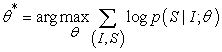(3-1)

Where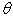is a parameter of the model, I is the input image, and S is its exact "translation" representation. Because S is the set of all statement outputs, its length is unlimited. So in this paper, we use the chain rule to model all the probabilities from S0 to SN. where N is this specific length, for example: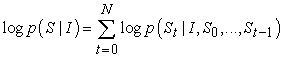(3-2)

Because the calculation is too complicated, this paper abandons the use of the parameter. During training, (S, I) is a training set 16, and we use stochastic gradient descent to optimize the sum of the probabilities of the entire training set (see Section 4 for further training details).

For the joint probability p(St|I, S0. For memory ht). The stored information is solved by using the following nonlinear function f after seeing a new input xt: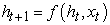(3.-3)

In order to more vividly illustrate the specific working principle of RNN, this paper designs two key selection problems: the specific form of f, and how to input pictures and output natural language sentences. For f, this paper uses an LSTM network, which performs very well on sequence tasks such as translation by current standards. This paper will describe this model (LSTM) in detail in the next section.

By using Convolutional Neural Networks (CNN), this paper accomplishes the task of representing images. At present, CNN has been widely used in the study of image tasks, and it is the latest technology in the field of target recognition and detection. The CNNs specifically chosen for this paper use a new batch normalization method. Furthermore, with transfer learning, they were shown to generalize to other tasks such as scene classification. These words are represented by an embedding model.

3.2. Sentence Composition System Based on LSTM

As shown in the above formula (3-3, the specific form of f depends on its ability to process image input and gradient descent, which is also the most important problem everyone faces when using CNN and RNN. In order to solve this problem, LSTM, This recurrent neural network with a special data structure was born, and has been successfully applied to various translation work and sequence generation tasks.

The core idea of the LSTM model is also its most innovative point in its storage unit c, which can encode the output sequence of each input (see Figure 24). Its execution instruction is issued by the "gate". If the value of the gate is 1, a value can be obtained from the gate control layer. If the value of the gate is 0, this value remains unchanged. All in all, the action of c is controlled by the following three gates: 1. Forget gate f, to control whether to inherit the cell content; 2. Input gate i, to control whether to read the input information; 3. Output gate o, to control whether to put new value output. The gate and cell changes and outputs are defined as follows 17: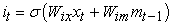(3-4)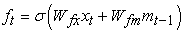(3-5)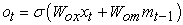(3-6)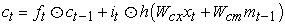(3-7)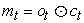(3-8)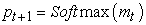(3-9)

Each W matrix is a training parameter. The product value of these AND gates can well avoid the phenomenon of gradient explosion or disappearance caused by weights greater than 1, thus enabling LSTM to perform high-intensity and large-scale training. Mt in the last equation is the input value of the function Softmax, which produces the distribution probability value Pt of all words.

• Figure 24. LSTM model diagram

As shown in FIG. The memory block of LSTM contains a storage unit c and three control gates. The blue line is used to indicate the circular connection - the output m at time t-1 is received by three gates and fed back to the memory c at time t, and in addition to the memory output m at time t, the output value of the previous time mt will be fed back to the Softmax function for further judgment.

Attention Mechanism originated from the study of human vision. In cognitive science, due to bottlenecks in information processing, humans selectively focus on a portion of all information while ignoring other visible information. The above mechanism is often referred to as the attention mechanism 20. For example, when people read, usually only a small number of words to be read are paid attention to and processed 18. In summary, the attention mechanism has two main aspects: deciding which part of the input needs to be focused on; and allocating limited information processing resources to important parts.

We can look at the Attention mechanism in this way (refer to Figure 25): imagine that the constituent elements in Source are composed of a series of <Key, Value> data pairs. At this time, given an element Query in Target, by calculating Query The similarity or correlation with each Key, the weight coefficient of each Key corresponding to the Value is obtained, and then the weighted sum of the Value is obtained, that is, the final Attention value is obtained. So in essence, the Attention mechanism is a weighted summation of the Value values of the elements in the Source, and Query and Key are used to calculate the weight coefficient of the corresponding Value 18. That is, its essential idea can be rewritten as the following formula: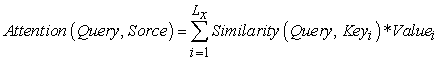(3-10)

Among them, Lx=||Source|| represents the length of Source, and the meaning of the formula is as described above. In the example of machine translation mentioned above, because in the process of calculating Attention, the Key and Value in Source are combined into one, pointing to the same thing, that is, the semantic code corresponding to each word in the input sentence ( RNN refers to the hidden layer state), so it may not be easy to see this structure that reflects the essential idea. Of course, from a conceptual understanding, Attention is still understood as selectively screening out a small amount of important information from a large amount of information and focusing on these important information, ignoring most of the unimportant information, this idea still holds 19. The process of focusing is reflected in the calculation of the weight coefficient. The greater the weight, the more focused on its corresponding Value value, that is, the weight represents the importance of the information, and the Value is its corresponding information.

• Figure 25. The essential idea frame diagram of the Attention mechanism

Another understanding can be drawn from the above figure, and the Attention mechanism can also be regarded as a kind of soft addressing (Soft Addressing): Source can be regarded as the content stored in the memory, the element is composed of the address Key and the value Value, there is currently a Key =Query query, the purpose is to retrieve the corresponding Value value in the memory, that is, the Attention value. Addressing is performed by comparing the similarity between Query and the address of the element Key in the memory. The reason why it is called soft addressing means that unlike general addressing, it only finds a piece of content from the storage content, but it may be possible from each Key address. The content will be taken out, and the importance of taking out the content is determined according to the similarity between Query and Key, and then the Value is weighted and summed, so that the final Value value, that is, the Attention value 20, can be taken out.

As for the specific calculation process of the Attention mechanism, if most of the current methods are abstracted, it can be summarized into two processes: the first process calculates the weight coefficient according to Query and Key, and the second process calculates the Value according to the weight coefficient. Weighted summation 21. The first process can be subdivided into two stages: the first stage calculates the similarity or correlation between the two according to Query and Key; the second stage normalizes the original score of the first stage; In this way, the calculation process of Attention can be abstracted into three stages 21 as shown in Figure 26 below.

• Figure 26. Schematic diagram of the three-stage calculation process of the Attention mechanism

In the first stage, different functions and calculation mechanisms can be introduced, and the similarity or correlation between the two can be calculated according to Query and a certain Key_i. The most common methods include: calculating the vector dot product of the two, calculating the The vector Cosine similarity is either evaluated by introducing an additional neural network 18, that is, the following formula: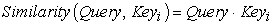(3-11)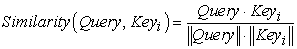(3-12)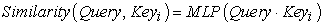(3-13)

The score generated in the first stage varies according to the specific method of generation, and its value range is also different. In the second stage, a calculation method similar to SoftMax is introduced to convert the scores of the first stage numerically. On the one hand, it can be normalized, and the original calculated scores can be sorted into a probability distribution where the sum of the weights of all elements is 1; on the other hand, it can also be the weights of important elements are more highlighted by the intrinsic mechanism of SoftMax 18. That is, it is generally calculated by the following formula:(3-14)

The calculation result of the second stage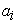is the corresponding weight coefficient, and then the weighted summation can be used to obtain the Attention value: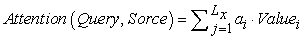(3-15)

Through the above three-stage calculation, the Attention value for Query can be obtained. At present, most of the specific attention mechanism calculation methods conform to the above-mentioned three-stage abstract calculation process.

3.4. Training Process

By training an LSTM model to predict each word in the sentence after seeing the image, and p(St|I, S0,…). In order to achieve these functions, we need to expand the LSTM - a LSTM memory for image input, and word input for each sentence, so that all LSTM models can share the same parameters and outputs. All periodic connections are transformed into unrolled versions of feedforward connections. In another easy-to-understand way, we use I to represent the input image, and a vector group S = (S0,...,SN) to represent each word of this image, and the expansion process is 17: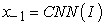(3-16)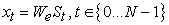(3-17)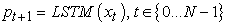(3-18)

Each feature vector St represents a word, and the dimension of each vector is equal to the size of the "thesaurus". It is worth noting that the first value S0 represents a special start word, while Sn represents a special stop word, which respectively represent the beginning and end of the sentence. It is worth noting that, by sending out the terminator Sn, the LSTM model receives the signal that the sentence has been constructed, thereby mapping both the image and the word into the same space. The image recognition adopts the convolutional neural network CNN, and the label word is embedded in We. The image I is input once and only once at t = −1, giving the LSTM model the input image content. After testing and experiments, inputting additional image content at each time step will lead to large errors, because the network will easily identify too many "details" in the image, resulting in overfitting. where the resulting loss is the sum of the negative log-likelihoods of the correct word at each time step 17, as follows: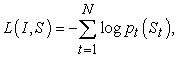(3-19)
3.5. Chapter Summary

This chapter mainly describes the specific construction of the overall model. First, CNN is used to extract image features, and the features are stored in the convolution kernel. Then, each feature is compared through a weighted calculation, and the feature vector with high comprehensive weight is selected for training. Find the corresponding labels in a centralized manner, and enter the LSTM for word sorting training. During the training process, the parameter values of the convolution kernels of the CNN are continuously adjusted through logistic regression and the LSTM "remembers" the word order of the output natural language sentences. In this way, a system model with the function of seeing pictures and talking through training is obtained, which is the result of the design of this project.

• Figure 27. LSTM work timeline expanded view

### 4. Training and Testing Experimental Results

4.1. Evaluation Indicators

Although it is sometimes unclear whether a description should be considered successful, several evaluation metrics have been proposed in the prior art. Obviously the most accurate method is to ask the rater to directly rate each output title for a given image, i.e. ask the rater to rate each generated sentence on a scale from 1 to 41. For this metric, this paper sets up an "Amazon Mechanical Turk" experiment. Each picture is graded by 2 students. The consensus level between the two is usually 65%. If there is disagreement, the scores are simply averaged and recorded as a score. For ANOVA, perform bootstrapping (replace and calculate mean/standard deviation for resampling results). Like it, the scores reported in this paper are greater than or equal to a set of predefined thresholds.

Although this metric has some obvious shortcomings, it has been shown to correlate well with human evaluations. In this work, this is also confirmed, as shown in Section 4.3. An extensive evaluation protocol, and the output produced by our system, can be found at http://nic.droppages.com/.

Based on the objective function in (1), complex models can be transcribed without BLEU. The choice about model selection and hyperparameter tuning is performed by the value of perplexity, but this paper does not report it, because BLEU always prefers 3. A more detailed discussion of metrics can be found in, and research groups studying this topic have reported other metrics deemed more suitable for evaluating titles.

In conclusion, the advantage of using proxies to describe is that known ranking metrics can be used, which is more convenient. On the other hand, converting a description generation task into a ranking task is not ideal: as the complexity of the image description increases, along with its dictionary, the number of possible sentences grows exponentially with the size of the dictionary, suitable for the prediction of new images. The number of defining sentences also goes down, and the potential computational complexity for efficient evaluation of the large number of sentences stored per image. The same is true in language recognition. Whether the sentence corresponding to a given acoustic sequence can be generated is the first problem to be solved. State-of-the-art methods for this task are now generative, producing sentences from a large dictionary.

Now that our model can generate descriptions of reasonable quality, and despite the ambiguity in evaluating image descriptions (where there may be multiple invalid descriptions), we believe that this paper should focus on generating evaluation metrics for the task rather than ranking.

4.2. Selection and Testing of Datasets

For evaluation, this paper uses a large dataset consisting of images and English sentences describing those images. The data set statistics are as follows:

With the exception of SBU, the sentences for each image are relatively visual and unbiased. SBU is the composition of the description given when the image is uploaded to Flickr. Therefore, the requirements of this performance cannot be guaranteed, and it can be seen that the data set has some interference. The Pascal dataset is traditionally only used for testing after the system has been trained on different data (such as any of the other four datasets), so it is not suitable as the first dataset for training. So we drop SBU, use the other 1000 images for testing, and use the rest for training. Use it to report results in the next section.

4.3. Results

Because the model in this paper is a data-driven, well-trained end-to-end system and is trained on a rich dataset, this paper hopes to answer questions such as "how does the size of the dataset affect generalization", "what kind of Transfer learning makes it happen" and "How to deal with weakly labeled examples". Therefore, we conduct experiments on 5 different datasets, which enable us to gain a deeper understanding of our model, as detailed in Section 3.2.

4.3.1. Training Process and Its Details

Many of the problems we encountered while training our models in this article were related to overfitting. The relatively large dataset of ImageNet is used for data-driven to solve the problem of difficult assignment and description.

Therefore, even with reasonably good results, the advantage of this method over the currently widely used engineering methods will only increase over the next few years as the size of the training set increases.

Nonetheless, this article considers several ways to deal with overfitting. The most obvious way to avoid overfitting is to initialize the weights of the CNN component of this system to a pre-trained model (e.g., on ImageNet). This paper does this in all experiments, and it does help a lot in generalization. Another set of weights that can be reasonably initialized is We, the word embedding. This paper attempts to initialize them from a large news corpus, but no significant effect is observed, and it is decided not to initialize them for simplicity. Finally, the paper employs some techniques to avoid model-level overfitting. Dropout and the overall model gave some improvements in BLEU scores, which are mentioned throughout the paper.

We train all weight sets using fixed learning rate and momentum-free stochastic gradient descent. All weights are initialized randomly except the weights of CNN, which are not changed in this paper because changing them would have more negative effects. This paper uses 512-dimensional embedding and LSTM memory size.

In addition, we perform basic tokenization preprocessing on the description, keeping all words that appear at least 5 times in the training set.

4.3.2. Generate Results

This paper reports the main results on all relevant datasets in Table 2 and Table 3 below. The state-of-the-art results of PASCAL and SBU do not use deep learning-based image features, so it can be said that this change alone can improve these scores by a large amount. The Flickr dataset has been used recently, but mostly for evaluation in retrieval frameworks. A notable exception is that they are retrieved and generated simultaneously and yield the best performance on the current Flickr dataset.

The human scores in Table 3 are calculated by comparing one of the "Human" titles with the other 4 "Human" titles. This paper does this for each of these five raters and averages their BLEU scores. Given that the BLEU score is calculated from 5 reference sentences instead of 4, this paper adds back the average difference of 5 reference sentences instead of 4 reference sentences to the score.

Given the significant progress the field has made over the past few years, this paper argues that it makes more sense to report on BLEU-4, the standard for moving forward in machine translation. Despite my constant efforts to get better test results, the model in this paper outperforms human raters. However, when human raters are used to evaluate the captions (i.e. when classmates are asked to give captions to the images), our model performs worse, suggesting that more work is needed to obtain better metrics. On the official test set (labels are only available through the official website), our model has a BLEU-4 score of 27.2.

4.3.3. Result Analysis

1) The tense grammar is wrong

As shown in the figure, this is an image of a football match, specifically Neymar in yellow jersey and two players in white jersey grabbing the ball. Due to the limitations of this model, only the most basic and most important ones will be generated. Description, that is, the content is:

Three guys are playing football.

Here is the result given by the program system:

• Figure 29. Schematic diagram of test results

Obviously not similar to the standard answer, but this will not affect the score too much, because there are many synonyms in the library. The bigger problem is the grammar, obviously there is a missing "is" here, which constitutes a grammar error, which probably won't produce a comprehension error in this picture, but will be greatly discounted in the scoring. Whether it's changed to "is playing" or just a simple present tense of "play" is correct in the evaluation. According to the analysis of the code and process, the possible problem is that there is an error in the feature vector extraction process of CNN, resulting in the use of "playing" during the process, and it is not considered to add "is" before. This is unnecessary when extracting the tense of the simple present tense. Perhaps a requirement should be set in advance that when the "-ing" tense occurs, the verb "be" is added before the word.

2) Anime image recognition error

• Figure 30. Schematic diagram of test animation picture results

This picture is not in MSCOCO's atlas, it is an image randomly selected from the Internet by this article to test the system's ability to recognize animation images.

Obviously there is a huge error in the recognition here. Whether it is recognizing cat as man or mouse as banana, it is a mistake that completely deviates from the meaning of the picture. After many verifications, the current recognition ability of this system for animation pictures is indeed true. There are huge flaws and no solution has been thought of at the moment. Because in the training process, the pictures in the data set used in this paper are all real pictures, so the system has no memory for the recognition of animation images. In addition, training by adding anime pictures is also proved to be infeasible. Because in the process of parameter setting through the Luo Ji regression function, the image of "cat" already has the setting memory of the real image. Since the difference between the animation image and the real image is too large, increasing the training set of the animation image will only cause greater recognition error.

In addition, due to the more complex factors such as style of animation in animation pictures, it also greatly increases the difficulty of training. So far, there is no good solution in this paper. It is hoped that this can be resolved in more in-depth research in the future. Fortunately, after the revision and adjustment of this article, the voice of the continuous tense has been able to be expressed correctly.

3) Black and white image recognition

It can be seen that the recognition of black and white images still has high accuracy, but there may be difficulties in the recognition of relatively small objects. For example, in this picture, "tea" or "foods" is mistakenly recognized as "cake" ". This is apparently due to the fact that the chromatic aberration of black and white photos is not as obvious as compared to color RPG pictures. Considering the pixel problem, even if it is artificial recognition, this article believes that there may be errors. For example, let this article identify it by itself. Under such clarity, it is difficult for this article to determine whether the content in this cup is wine or tea, which may need to pass through It is obvious that the system in this paper has not yet reached such a high level, and is even far from such a goal.

Considering it comprehensively, this article still regards it as a successful case (except for the tense grammar, which has been revised later).

• Figure 31. Schematic diagram of the test results of black and white pictures

4) Complex images

• Figure 32. Schematic diagram of the result of testing complex pictures

Obviously, it is still difficult for the system to recognize images with too complex elements or too much content. Although the MSCOCO data set with relatively rich content has been used for training, the discriminative ability is obviously not enough to recognize such complex images. , you can see that the title given by the system is very outrageous. However, in this aspect, this paper believes that there will be greater improvements after training with more complex and comprehensive datasets. Due to computer performance and network reasons, this article cannot be improved in a short time, but this is not an unsolvable problem. This article believes.

4.4. Summary of This Chapter

The above examples are some categories of images that the system is more likely to generate errors after sorting out this article. In addition, most of the images are recognized without major problems, which are also in line with the expected goals of this article. Some of the problems that this paper can improve or solve in a short period of time have been improved and dealt with (such as temporal grammar description ability). Some other problems are still difficult to solve at present, and may need to be solved in this article after in-depth study and research, and under better equipment conditions, but in any case, the current performance and indicators have fully reached the expected value of this article, and also achieved The basic purpose of this subject.

### References

  Liu Siyang. Research on Natural Language Reasoning Based on Sequence-Tree Encoder Fusing Syntactic Information [D]. Zhejiang University, 2018. In article  Guo Jimin. Research and implementation of object recognition method based on deep neural network [D]. University of Electronic Science and Technology of China, 2018. In article  Zhang Yanqi. Image Chinese semantic understanding based on deep learning [D]. Harbin Institute of Technology, 2017. In article  Ma Xinrui. Research on Image Recognition Algorithm Based on Primitive Feature Analysis [D]. Xidian University, 2019. In article  Song Jiantao. Research and implementation of early warning system based on agricultural product traceability platform [D]. Beijing University of Technology, 2018. In article  Han Guo Feng. Personalized recommendation algorithm for tourist attractions based on transfer learning [D]. Shaanxi University of Science and Technology, 2019. In article  Guo Fei. Research on target detection of mechanical parts based on deep learning [D]. Lanzhou University of Technology, 2019. In article  Chen Jiaming. Research and simulation of satellite positioning error compensation technology based on convolutional neural network [D]. Beijing University of Posts and Telecommunications, 2018. In article  Liu Lei. Application of Tensorflow-based Recurrent Neural Network Model in Air Quality Prediction in Shanghai [D]. Shanghai Normal University, 2019. In article  Gao Maoting, Xu Binyuan. Recommendation algorithm based on recurrent neural network [J]. Computer Engineering, 2019, 45(08):198-202+209. In article  Wu Haoyu. Research and Application of Text Description Generation Image Algorithm Based on Generative Adversarial Network [D]. Nanjing Normal University, 2019. In article  Fu Yuan. A method and system for testing RDMA data transmission on Tensorflow software [J]. Information Communication, 2019(08): 137-138. In article  Yin Yuecheng. Experimental research on turning of DT4E pure iron materials [D]. Dalian University of Technology, 2019. In article  Lv Ruru. Research on original information collection and processing system of digital copier [D]. Nanjing Forestry University, 2011. In article  Xie Pengfei. Geostatistical inversion method based on deep learning [D]. Yangtze University, 2019. In article  Zhi Shuaifeng. Research on 3D object recognition technology based on convolutional neural network [D]. National University of Defense Technology, 2017. In article  Dou Min. Design and implementation of video semantic analysis system based on CNN and LSTM [D]. Nanjing University of Posts and Telecommunications, 2018. In article  Yang Xiaochun, Hou Jixiang, Zheng Han, Wang Bin. An image description generation method based on multiple attention mechanisms and external knowledge [P]. Liaoning Province: CN112784848A, 2021-05-11. In article  Ge Hongwei, Yan Zehang. An automatic image caption generation method based on multimodal attention [P]. Liaoning Province: CN108829677B, 2021-05-07. In article  Hu Fei, Peng Liang, Zhong Wei, Fang Li, Ye Long, Zhang Qin. Object detection method, device and medium based on image and category attention [P]. Beijing: CN112733944A, 2021-04-30. In article  Zhou Yiwen. Research on Question Answering System for Legal Field [D]. Hunan University, 201. In article

Published with license by Science and Education Publishing, Copyright © 2022 Kexin Ye, Lei Zhang and Tianran LiThis work is licensed under a Creative Commons Attribution 4.0 International License. To view a copy of this license, visit http://creativecommons.org/licenses/by/4.0/